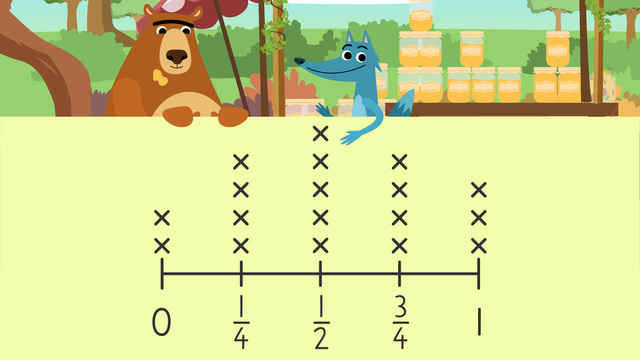# Line PlotsRate this video

Ø 5.0 / 1 ratings

The authorTeam Digital
Line Plots
CCSS.MATH.CONTENT.4.MD.B.4

## Information about the videoLine Plots

### Contents

#### What are line plots?

The line plot definition is a graph that displays data as points or x’s above a number line, showing the frequency of each value. Line plot data changes from lists to an organized diagram.#### In this Video

In this line plots video, we learn about using line plots with fractions. Nari has set up a honey sale at his Sticky Fingers Honey Company stand. He notices that honey is missing from some jars and must take inventory to know what he has available. He collects a line plot data set of how many jars have each level of honey. Then he uses line plots and fractions to create a line plot diagram that easily shows him how much he has in each category. In this line plots lesson, we can see how organizing data helps us easily answer questions about the data collected. In this line plots video 4th grade, we will find out if Nari can save the sale!

#### Line Plot Examples

Here are some line plots for 4th grade examples of information learned. Line plots 4th grade focuses on line plots fractions and we can use this information to answer questions about the data.#### Follow Up Line Plots Activities

Following the video, there is line plots practice and line plots worksheets.

### TranscriptLine Plots

"This sale is going to be amazing! “Hmmm…..what in the world?" “The sale is going to start soon and I don’t know how much honey is in every jar!” Nari needed to get to the bottom of this mystery and decided to record the amounts of honey in each jar. Because Nari’s list of honey amounts is disorganized, it's not a useful way to look at the information. We can help Nari save the honey sale by organizing his data into a line plot. "Line Plots" A line plot is a graph that displays data along a number line in a way that organizes the frequency of the information. We can take Nari's list and create a line plot that will organize the honey inventory he has to sell. First, let’s organize the list of honey jars by grouping the like fraction amounts together. This list represents our DATA SET. What numbers will we include on our number line? We will include all the numbers from our data set in order from least to greatest. Make sure they are spaced an equal distance apart. Now we’ll need to label these units of measurement used for the number line. These fractions represent how much of the jar is filled with honey, so we will label this LEVELS OF HONEY. Where do we get the information for making the plots on our line? We use the data set to tell us how many we have of each kind. The next step is to plot the data points on the line. We will be using x's to stand for the jars of honey. Nari has two jars that are empty, so we will make two x’s above the zero on the number line. In order to stay organized, the x’s need to be the same size and an equal distance apart. How many jars are filled one-fourth the way up? "There are four jars filled to one-fourth, so we can make four x's HERE to represent these jars." How many one-half fractions are on the list? Five (...)so there are five jars that are half full. We’ll add those plots to our number line. Continue on counting the number of three-fourths fractions from our data set and make x’s on the graph. How many jars are three-fourths full? Four.(...) so we have made four x’s above the number line. Finally, count and make the x’s for the full jars. The final step is to title the line plot so everyone knows what information this graph presents. What information do we learn from these line plots? We learn about Nari’s honey jar inventory for his sale, so we will title this STICKY FINGERS INVENTORY. Now that we have organized Nari’s inventory of honey jars, we can easily answer questions about what he has available to sell. Which quantity does Nari have the most of? Nari has the most of the jars that are filled halfway. Which quantities have the same amount of jars to sell? There are four jars of BOTH one-fourth and three-fourths levels. How many more full jars are there than empty ones? One, because there is one more x above the one on the line plot than there is above the zero. Finally(...)How many total jars of honey does Nari have to sell? To solve this problem you add up ALL the x’s above one-fourth,(...) one half, (...) three-fourths, and one whole. Nari has SIXTEEN jars of honey! Four, plus five, plus four, plus three equals sixteen. Why did we not count the x’s above the zero? Nari would not sell EMPTY jars! That would be silly! Remember(...) The purpose of a line plot is to show data in an organized way so we can answer questions about the information. First, we create a number line that includes all the measurement values from our data set. Next, we will plot the data by making x’s above the measurement that match the number of times the data is given. Then, label the units of measurement for the line plot. Finally, title your graph that tells EXACTLY what information the line plot shares. " Hey Nari, (...)Where'd all the DELICIOUS honey go?" "GUS!! (...) WAIT! (...)YOU'RE the one behind all of THIS?!"